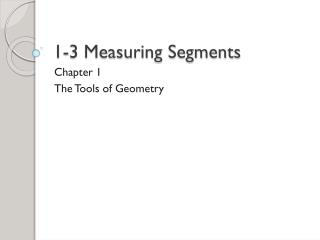DownloadDownload Presentation1-3 Measuring Segments

# 1-3 Measuring Segments

Télécharger la présentation## 1-3 Measuring Segments

- - - - - - - - - - - - - - - - - - - - - - - - - - - E N D - - - - - - - - - - - - - - - - - - - - - - - - - - -
##### Presentation Transcript

1. 1-3 Measuring Segments Chapter 1 The Tools of Geometry

2. Last class we worked on new vocabulary and 3 postulates • You may use • Your notes from class • Your Lesson Closure Book …to complete the assignment 1-2 Practice. • We will check this assignment in 30 minutes and turn it in.

3. Today’s Lesson: Measuring Segments The Burbot fish are found in Europe, Asia, and North America (the Great Lakes). Adult Burbots grow to be between 30 and 60 cm. This shows a young fish. How would you determine its length to the nearest centimeter? Use this scale

4. Postulate 1-5 Ruler Postulate • The real number that corresponds to a point on a number line is the coordinate of the point.

5. Find the measure of each segment. • What is DF? Use the Ruler Postulate • What is AD? • What is BD? • What is BF?

6. Postulate 1-6 Segment Addition Postulate • If three points, A, P, and B are collinear and P is between A and B, then AP + PB = AB.

7. 3x + 8 2x + 4 L M N • If LN = 32, what are LM and MN?

8. 4x + 6 7x + 15 J K L You try this one • If JL = 120, what are JK and KL?

9. Comparing Segment Lengths • When numerical expressions have the same value, we say they are equal. 3 + 7 = 4 + 6 • If two segments have the same length, we say they are congruent segments. Symbol: ≅

10. Comparing Segment Lengths Are AC and DF congruent? Are AC and CD congruent?

11. Comparing Segment Lengths Point C is equidistant from both A and D. Therefore it is the midpoint of AD. Midpoint: a point that divides a segment into two congruent segments.

12. Any point, line, or ray that intersects a segment at its midpoint is said to bisect the segment. • That point, line, ray, or segment is called a segment bisector.

13. We can use the midpoint to find solutions to problems. T S R • S is the midpoint of RT. • What are RS and ST? • What is RT? 7x – 3 3x + 1